# Weak Interaction

(redirected from Weak decay)
Also found in: Dictionary, Thesaurus.

## weak interaction

[′wēk ‚in·tər′ak·shən]
(particle physics)
One of the fundamental interactions among elementary particles, responsible for beta decay of nuclei, and for the decay of elementary particles with lifetimes greater than about 10-10 second, such as muons, K mesons, and lambda hyperons; it is several orders of magnitude weaker than the strong and electromagnetic interactions, and fails to conserve strangeness or parity. Also known as beta interaction.
McGraw-Hill Dictionary of Scientific & Technical Terms, 6E, Copyright © 2003 by The McGraw-Hill Companies, Inc.
The following article is from The Great Soviet Encyclopedia (1979). It might be outdated or ideologically biased.

## Weak Interaction

one of the four known fundamental interactions between elementary particles, the other three being the electromagnetic, gravitational, and strong interactions. The weak interaction is much weaker than the strong and electromagnetic interactions and is much stronger than the gravitational interaction.

Characteristics. The strength of an interaction can be assessed from the rates of the processes that the interaction brings about. The rates of processes are usually compared with each other at energies of the order of 108— 109 electron volts (eV). Such energies are characteristic of elementary particle physics, since the masses, in energy units, of most elementary particles are of precisely this order of magnitude—for example, the mass of the pion, or π-meson, is 1.4 × 108 eV, and the mass of the proton is 9.4 × 108 eV. At such energies, a process due to the strong interaction occurs in ~10-24sec; during this time, a hadron, or strongly interacting particle, moving at a speed of the order of the speed of light (3 × 1010 cm/sec) traverses a distance of the order of the hadron’s dimensions (~ 10-13 cm). An electromagnetic process under the same conditions lasts approximately 10-21 sec. Weak processes, or processes occurring as a result of the weak interaction, have a much greater characteristic time: ~ l0-10 sec. Thus, in the world of elementary particles weak processes are extremely slow.

Another characteristic of an interaction is the mean free path of a particle in matter. Strongly interacting particles are usually retarded by an iron plate a few tens of centimeters thick. By contrast, a neutrino, which has only the weak interaction, could pass through an iron plate a billion kilometers thick without undergoing a single collision. The gravitational interaction is even weaker. At an energy of ~ 109 eV, it is 1033 times weaker—that is, weaker by 33 orders of magnitude—than the weak interaction. In everyday life, however, the role of the gravitational interaction is much more prominent than the role of the weak interaction. The reason for this circumstance is that the gravitational interaction, like the electromagnetic interaction, has an infinite range. Consequently, a body on the earth’s surface experiences the gravitational attraction of all the atoms of which the earth consists. By contrast, the range of the weak interaction is so short that it has not yet been measured. It is surely less than 10-14 cm and may be less than 10-15 cm, a value that is two orders of magnitude less than the range of the strong interaction. As a result, the weak interaction between, for example, the nuclei of two adjacent atoms 10–8 cm apart is negligible.

Nonetheless, despite its small magnitude and short-range character, the weak interaction plays a very important role in nature. If it were possible to “turn off” the weak interaction, the sun would be extinguished, since the process of the transformation of a proton (p) into a neutron (n), positron (e+), and neutrino (v) would be impossible. The “burning” of hydrogen in the sun occurs through this process: four protons are transformed into a helium nucleus consisting of two protons and two neutrons. This process constitutes the source of the energy of the sun and most of the stars. Weak processes involving the emission of a neutrino in general appear to play an exceptionally important role in the evolution of stars. Such processes, for example, may account for energy losses by very hot stars and for the mechanisms of supernova explosions involving the formation of pulsars. Another illustration of the importance of the weak interaction is presented by the muons (μ), π-mesons, and strange particles. If the weak interaction did not exist, the particles of these three types would be stable and would be widely encountered in ordinary matter. In actuality, strange particles, for example, decay through the weak interaction into non-strange particles in millionths or billionths of a second.

The weak interaction has such an important role because it does not obey a number of prohibitions that are obeyed by the strong and electromagnetic interactions. In contrast to the strong and electromagnetic interactions, the weak interaction violates the law of conservation of strangeness. The weak interaction also violates another fundamental symmetry of nature—reflection symmetry (seeSPACE INVERSION); weak decays maximally violate the law of conservation of space parity and of charge parity (seeCHARGE CONJUGATION). Some weak decays of long-lived neutral kaons, or K-mesons, violate the conservation of combined parity (seeCOMBINED INVERSION) and the conservation of the time reversibility of microprocesses (seeTIME REVERSAL). The relative frequencies of such decays are tenths of a percent. (See below for further details.)

The intensities of weak processes increase rapidly with energy. For example, the energy released in the beta decay of a neutron, ~ 1 million electron volts (MeV), is small compared with energies of the order of the rest energy of hadrons; a free neutron has a lifetime of about 103 sec, which is 13 orders of magnitude greater than the lifetime of the Λ-hyperon. The cross section for interaction with nucleons (protons and neutrons) is approximately 1 million times greater for neutrinos having energies of ~ 100 gigaelectron volts (GeV) than for neutrinos with energies of ~ 1 MeV. It is not yet known to what energies the cross section continues to increase with increasing energy. The cross-section growth may continue up to energies of ~ 1,000 GeV in the center-of-mass system of the colliding particles. On the other hand, the increase in the cross section may cease at much lower energies.

Beta decay; Fermi’s theory. The most widely encountered weak process is the β-decay of radioactive atomic nuclei. The phenomenon of radioactivity was discovered in 1896 by A. H. Becquerel. During the first third of the 20th century the energy spectra of β-radioactive nuclei were experimentally investigated by such scientists as E. Rutherford, J. Chadwick, and L. Meitner. A result of this research was the hypothesis advanced in 1931 by W. Pauli that, in addition to an electron (e-), another light particle, now known as the neutrino, is emitted in a β-decay. Although the free neutrino was not experimentally detected until 1956, E. Fermi in 1934 put forth, on the basis of Pauli’s hypothesis, a seminal theory of β-decay. With some modifications, Fermi’s theory underlies the present-day theory of the weak interaction.

According to Fermi’s theory, the electron and neutrino (more accurately, the antineutrino) that are emitted by a β-radioactive nucleus do not exist prior to the decay but appear at the moment of decay. This phenomenon is analogous to the emission of low-energy photons (visible light) by excited atoms or of high-energy photons (γ-quanta) by excited nuclei. Light is known to be emitted by an electron when the electron undergoes a transition from one atomic level to another, lower level. In much the same way, γ-quanta are emitted by nucleons that move from higher, excited levels in a nucleus to lower levels. The interaction of the electric charges with the electromagnetic field is the principal cause of these processes. The moving charged particle—an electron or proton—disturbs the electromagnetic field, and the energy of the particle is transferred to field quanta, or photons. The moving charge creates an electromagnetic current, and we usually speak of the interaction of the photons with the electromagnetic current. In quantum electrodynamics, the interaction between an electron and a photon is described by an expression of the form

eψ̄ψA

Here, e is the elementary electric charge, which is the electromagnetic coupling constant (the strength of the electromagnetic interaction is characterized by the dimensionless constant α= e2/ħc≈ 1/137, where ħis Planck’s constant and c is the speed of light), ≈ ψ is the annihilation operator for the electron in the initial state, ψ̄ is the creation operator for the electron in the final state, and A is the photon creation operator. Thus, instead of the initial electron two particles appear: an electron in another state (of lower energy) and a photon.

The interaction between an electron and a photon is described more accurately by the expression

(1) eψ̄γμψAμ

The subscript μ in the quantity Aμ takes on the four values 0, 1, 2, and 3 and indicates that the quantity Aμ is transformed as a four-dimensional vector under Lorentz transformations. It may be recalled that a four-vector is formed, for example, by the four-dimensional coordinates of the particle xμ (x0 = ct, x1 = x, x2 = y, and x3= z) or by the particle’s energy and momentum pμ (p0 = E/c, pl = px, p2 = py, and p3 = pz, where E is the particle’s energy and px, py, and pz are the components of the particle’s three-dimensional momentum). The scalar product of the two four-vectors is given by the equation xμpμ = ×0p0 – ×1p1 – x2p2 – ×3p3 The summation is carried out over identical subscripts μ; for brevity, the summation sign is omitted. Since the electromagnetic field is a vector field, the quantum of this field—the photon—is spoken of as a vector particle. The quantity ψ̄γμψ is called the electromagnetic current. In order for interaction (1) to be Lorentz-invariant, the electromagnetic current ψ̄γμψ must also be a four-vector, and the interaction between the current and the photon field must be given by the scalar product of two four-vectors—the repetition of the subscript μ in (1) indicates that the interaction is such a scalar product. The four Dirac matrices γμ are introduced so that the electromagnetic current four-vector can be constructed from the operators ψ̄ and ψ, which are four-component spinors with respect to Lorentz transformations.

The significance of the operators ψ̄ and ψ can now be indicated more precisely. These operators describe not only processes involving particles (electrons) but also processes involving antiparticles (positrons). The operator ψ annihilates an electron or creates a positron, and the operator ψ̄ creates an electron or annihilates a positron. The operator A describes both the creation and the annihilation of photons, since the photon, as a truly neutral particle, is its own antiparticle. Thus, the interaction eψ̄ψA describes not only the emission and absorption of light by electrons and positrons but also such processes as the production of electron-positron pairs by photons or the annihilation of such pairs with the resulting creation of photons. The exchange of a photon (γ) between two charged particles leads to the interaction of these particles with each other. For example, the scattering of an electron by a proton may occur; such a scattering event can be schematically represented by a Feynman diagram, as in Figure 1,a. When a proton in the nucleus under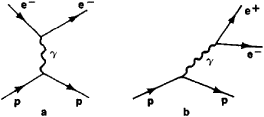Figure 1

goes a transition from one level to another, the same interaction can result in the production of an electron-positron pair by the nucleus (Figure 1,b).

Fermi’s theory of β-decay is in fact analogous to the theory of electromagnetic processes. Fermi based his theory on the interaction of two weak currents. These currents, however, interact with each other through contact rather than at a distance through the exchange of a particle that is the field quantum (the photon in the case of the electromagnetic interaction). In present-day notation, this contact interaction has the form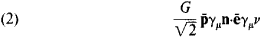Here, G is the Fermi, or weak-interaction, constant, whose experimental value is G ≈ 10-49 erg-cm3. The quantity G/ħc has the dimension of length squared; in units c = ħ = 1, G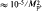. where Mp is the mass of the proton. As for the other symbols in (2), p̄ is the proton creation (antiproton annihilation) operator, n is the neutron annihilation (antineutron creation) operator, ē is the electron creation (positron annihilation) operator, and v is the neutrino annihilation (antineutrino creation) operator. (Here and below the particle creation and annihilation operators are designated by the symbols of the corresponding particles in boldface type.) The current P̄γμn, which transforms a neutron into a proton, subsequently came to be known as the nucleonic, or nucleon, current, and the current ēγμv was termed the leptonic, or lepton, current (the electron and neutrino are leptons). Fermi postulated that weak currents, like the electromagnetic current, are four-vectors. A Fermi interaction is therefore called a vector interaction. (Fermi’s original notion was that the nucleonic current P̄ ēγμn is analogous to the electromagnetic current P̄γμp and that the leptonic current ēγμv is analogous to the electromagnetic field Aμ.) The nucleonic and leptonic currents, however, had equal standing in the expression as he wrote it, and the subsequent development of the theory increasingly emphasized this equal standing.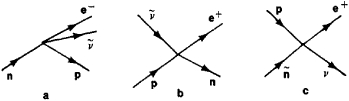Figure 2

Like the emission of an electron-positron pair, the β-decay of a neutron can be described by a diagram (Figure 2,a). (In the present article, antiparticles, with the exception of positrons, are indicated by a tilde [̃] above the symbols of the corresponding particles.) It follows from what has been said regarding particle creation and annihilation operators that the interaction of leptonic and nucleonic currents should also produce other weak processes—for example, the reaction ṽ + p → e+ + n (Figure 2,b) and the annihilation of pairs, as in p + ñ → e++ v (Figure 2,c) and P̄ + n → e- + ṽ.

An important difference between a weak current and an electromagnetic current is that the weak current changes the charge of particles, but the electromagnetic current does not. Thus, a weak current transforms a neutron into a proton and transforms an electron into a neutrino, but the electromagnetic current leaves a proton a proton and leaves an electron an electron. For this reason, the weak currents p̄n and ēv are called charged currents. According to this terminology, the conventional electromagnetic current ēe is a neutral current. (Neutral weak currents of the type v̄μ vμ andμ̄μ are discussed below.)

Fermi’s theory was based on the results of investigations in three different areas: experimental studies of the weak interaction proper (β-decay), which led to the hypothesis of the existence of neutrinos; experimental research on the strong interaction (nuclear reactions), which led to the discovery of protons and neutrons and to the understanding that nuclei consist of these particles; and experimental and theoretical investigations of the electromagnetic interaction, which laid the foundation for quantum field theory.

The subsequent development of elementary particle physics, especially in recent years, has repeatedly shown the interrelation of investigations of the strong, weak, and electromagnetic interactions to be very fruitful.

For over 20 years an important concern of theoretical and experimental research was the question of whether the weak β-decay interaction is a vector interaction. During this period, the weak interaction was found to be responsible not only for the β-decay of nuclei but also for slow decays of unstable elementary particles. After the discovery of muons, pions, kaons, and hyperons in the late 1940’s and early 1950’s, a hypothesis of the universal character of the interaction causing the decays of these particles was formulated.

Parity violation. In 1956, Tsung Dao Lee and Chen Ning Yang of the USA advanced, in a theoretical investigation of Kmeson decays, the hypothesis that the weak interaction does not conserve space parity. The nonconservation of parity was soon detected experimentally in the decays of several particles. For example, Chien-Shiung Wu and co-workers, of the USA, found nonconservation in the β-decay of nuclei. The Americans R. Garwin and L. Lederman and other scientists detected nonconservation of parity in the decay of the muon.

In the fall of 1956, L. D. Landau and, independently, Lee, Yang, and R. Ohme advanced the hypothesis that the weak interaction violates not only space parity (P) but charge parity (C) as well. The violation, however, should occur in such a way that the product of the two—combined parity (CP parity)—is conserved. The invariance of the weak interaction with respect to combined inversion would mean that processes involving particles were “mirror images” of the processes involving the corresponding antiparticles. For example, the angular distribution of the electrons (e-) in the decay of negative muons (μ-) and the angular distribution of the positrons (e+) in the decay of positive muons (μ+) should be as in Figure 3. Violations of combined inversion have been observed, but only in decays of neutral Kmesons (see below).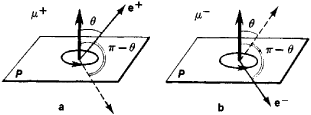Figure 3. The violation of space parity and charge parity in weak processes and the invariance of the weak interaction under combined inversion, as illustrated by two decays: (a) the decay μ+ → e+ + v0 + ṽμ, (b) the decay μ- → e- + ṽe + vμ. The boldface arrow indicates the direction of the spin of the muon μ+ or μ-; the “circle” with an arrowhead shows the direction of “rotation” corresponding to the indicated direction of spin. The light arrow shows the direction of the momentum of the positron e+ or electron e- The broken arrow represents the emission of e+ or e- under space reflection (the direction of spin—the direction of “rotation”—does not change under reflection). If space parity were conserved in weak interactions —that is, if reflection symmetry existed—then the probabilities of the emission of e+ or e- at the angles θ and π — θ to the direction of the spin of μ+ or μ. would be identical. If the weak interaction were invariant with respect to charge conjugation, then the decays of μ+ and μ- would look the same. In actuality, this situation is not observed. The invariance of weak interactions under combined inversion is manifested in the following: the probability of the emission of e+ at the angle θ to the spin of μ+ (a) is the same as the probability of the emission of e- at the angle π — θ to the spin of μ- (b).

V-A theory. The numerous experimental data were generalized by M. Gell-Man, R. Feynman, R. Marshak, and E. Sudarshan of the USA in 1957 in the theory of the universal weak interaction, or V-A theory. Here, as in Fermi’s theory, the weak interaction occurs through weak currents. The two theories differ in only two points.

First, in Fermi’s theory the weak current is a vector current, whereas in the new theory the current consists of a vector part (V) and an axial vector part (A). (An axial vector current is constructed by means of the matrices γμ γ5 where γ5 = iγ0γ1γ2γ3.) Under Lorentz transformations, both the vector and axial vector currents behave identically, like conventional four-vectors.

Under reflections, however, vector and axial vector currents behave differently, since they have different parity. As a result, the weak current does not have a definite parity. This property of the weak current is reflected in the experimentally detected nonconservation of parity in the weak interaction.

Second, the current in the new theory has, in addition to the terms p̄n and ēve, two other terms—a muonic current and a strangeness-changing hadronic current. The muonic current, μ̄vμ, transforms a muon neutrino vμ into a muon. (The muon neutrino was experimentally discovered in 1962, and the neutrino occurring in reactions together with the electron [positron] came to be called the electron neutrino, symbolized ve.) The strangeness-changing hadronic current is responsible for the decay of strange particles (K-mesons and hyperons). The nucleonic current p̄n in the new theory becomes what is known as the strangeness-conserving hadronic current.

Hadronic currents (strangeness-changing and strangeness conserving) are more complex than leptonic currents, since the number of known leptons is small (e±, ve, ṽe, μ±, vμ, ṽμ) and the number of known hadrons reaches several hundred. It may be assumed, however, that all known hadrons are built up of more elementary particles called quarks—the proton quark p, the neutron quark n, the strange quark γ, and the corresponding antiquarks. The nucleons consist of three quarks: p = ppn and n = nnp. The ∧-hyperon contains, in addition to the p- and n-quarks, the strange quark: Λ = pnλ. Mesons consist of a quark and an antiquark: π+ = pñ, π- = p̃n, K+ = p λ-, K- = λ, K0 = nλ̃, and K̃0 = ñ̃λ.

The quark hypothesis well accounts for a broad range of phenomena pertaining to the properties of strong and electromagnetic interactions of hadrons and to the classification of hadrons. According to this hypothesis, the β-decay of a neutron occurs when one neutron quark in a neutron is transformed into a proton quark with the emission of the pair e- and ṽe. Similarly, the decay Λ → p + e- + ṽe occurs through the transformation of a λ-quark into a p-quark: λ → p + e- + ṽe. The weak hadronic current here can be written in the form

(3) P̄ncos θ + p̄λsin θ

where P̄ is the p-quark creation (P̃-quark annihilation) operator, n is the n-quark annihilation (ñ-quark creation) operator, A is the λ-quark annihilation (λ̃-quark creation) operator, and θ is the Caoibbo angle. Experiment has shown θ to be equal to approximately 15°. It is evident that sin θ < cos θ. The significance of this fact is that decays involving a change in the strangeness of particles are suppressed—that is, occur with a lower probability—relative to decays in which strangeness is conserved. For example, the decay Λ→ p + e- + ṽe is suppressed by comparison with the neutron decay n →p + e- + ṽe. This assertion regarding relative probability should not be taken too literally, however, since the probability of a decay is determined not only by the strength of the interaction of the corresponding currents but also by the amount of energy released in the decay. The probability is proportional to the fifth power of this energy. The energy released in the decay of a neutron is two orders of magnitude smaller than the energy released in the decay of a Λ-hyperon. Thus, the smallness of sin θ means only that although the Λ-hyperon decays ten orders of magnitude faster than the neutron, the Λ-hyperon decays approximately 20 times slower than the neutron would decay if it had the mass of the Λ-hyperon. If the total weak current is designated by jw so that

(4) jw = ēve + μ̄vμ + P̄ncos θ + p̄λsin θ

then the energy (or more accurately, the Lagrangian density L) of the weak interaction acquires the form: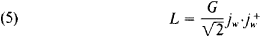Here, G is the Fermi constant, and the superscript + indicates the Hermitian adjoint current: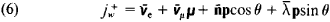Each of the terms in the currents jw and jw+ represents the sum of a vector and an axial vector—for example, ēγμ(1 + γ5)ve. Such an expression for the weak interaction describes all known weak processes except the neutral currents (see below). The processes to which the expression applies can be classified according to the terms in jw and jw+ that couple to generate them. Each process, which represents the product of a term in jw and a term in jw+, is indicated by a number in Table 1. Direct processes and the corresponding inverse processes are located at positions in the table that are symmetric with respect to the diagonal.

Table 1. Weak processes
ēveμ̄vμn̄pλ̄p
e e ....................1234
μμ .....................2567
p̄n .......................3689
p̄λ .......................47910

The number 1 in Table 1 represents the current product (v̄e e)(ēve), which describes the elastic scattering process ve + e → ve + e. This process has not yet been observed, but the accuracy of the experiments used is less than that necessary to measure the theoretically predicted magnitude.

The number 2 represents the current product (v̄e e)(μ̄vμ), which is responsible for the decays μ+ → e+ + ve + ṽμ and μ- → e- + ṽe + vμ. These decays have been well studied and are excellently described by theory.

The current product (P̄n)(ēve)—the number 3—is responsible for the β-decays of nuclei and for decays of the type π+ → e+ + ve and π+ → π0 + e+ + ve. The probability of the second decay was predicted by Ia. B. Zel’dovich and S. S. Gershtein in 1955 on the basis of the analogy between the weak vector current and the electromagnetic current (on the basis of the hypothesis of the conserved vector current); subsequent experiments confirmed this prediction. This interaction also leads to the neutrino reaction ṽe+ p → e+ + n, which was detected in 1956 by F. Reines and C. Cowan of the USA in an antineutrino beam from a nuclear reactor.

The current product (P̄λ)(ēve)—the number 4—is responsible for β-decays of strange particles, where the strangeness changes—for example, Λ→ p + e- + ṽe, Σ- → n + e- + ṽe, K+ → e+ + ve, and K+ → e+ + ve + π0. These decays are characterized by three selection rules that follow from the type of the weak current and that have been experimentally confirmed: first, ∆S = ± 1, where ∆S is the change in the strangeness of the hadrons participating in the decay (∆S = S1 - S2, where S1 is the strangeness of the decaying hadron and S2 is the strangeness of the hadrons appearing as a result of the decay); second, ∆S = ∆Q, where ∆Q is the change in the electric charge of the hadrons; third, ∆T = 1/2, where ∆T is the difference between the hadron’s isotopic spins in the initial and final states.

The number 5 represents the current product (v̄μμ)(μvμ). The process involved here is vμ → μ+ + μ- + vμ which should occur when a high-energy neutrino interacts with the Coulomb field of the nucleus. The theoretically predicted value of the cross section for this process is less than the limit attained in the experimental search for the process.

The current product (P̄n)(μ̄vμ)—the number 6—is responsible for processes of the capture of muons by atomic nuclei. Underlying such processes is the reaction μ- + p → n + vμ. This capture has been studied in detail for a large number of different nuclei. The product is also responsible for the primary channel of the decay of charged π-mesons (π+ → μ+ + vμ and π- → μ- + ṽμ). In addition, the product is responsible for most high-energy neutrino reactions observed in neutrino beams formed by the decay of the π- and K-mesons that result when nuclei are bombarded with energetic protons from accelerators. Such neutrino beams can be produced in a number of laboratories. When an energetic neutrino collides with a nucleon, there can occur the quasi-elastic processes vμ + n → μ- + p or ṽμ + p → μ+ + n and the inelastic processes vμ(ṽμ) + nucleon → μ-+) + nucleon + mesons. In conventional high-energy neutrino beams the fraction of electron neutrinos is small, since π-mesons principally decay with the emission of μ and vμ.

The current product (P̄λ)(μ̄vμ)—the number 7—leads to muon decays of strange particles with a change in strangeness. Examples are Λ → p + μ- + ṽμ, Σ → n + μ-+μ + K+ → μ+ + vμ, and K+ → μ+ + vμ + π0. Such decays obey the same selection rules as the corresponding electron decays (number 4). This product is also responsible for neutrino reactions in which single strange particles are produced.

The number 8 represents the current product (P̄n)(n̄p), which leads to weak nuclear forces that, in contrast to ordinary nuclear forces, do not conserve space parity (P). Such P-odd forces, which were theoretically predicted, have been experimentally detected by such Soviet scientists as Iu. G. Abov, P. A. Krupchitskii, V. M. Lobashev, and V. A. Nazarenko.

The product (p̄λ)(n̄p)—the number 9—is responsible for multiparticle nonleptonic decays of strange particles: Λ → p + π- Σ+ → n + π+, Ξ- → Λ + π-, Ω-→ Λ + K-, Ω- → Ξ- + π0, K0 → π + π-, + K+ → π+ + π+ + π-. In all these decays, ∆S = ± 1, and ∆T = 1/2.

The product (P̄λ)(λ̄p)—the number 10—contributes to P-odd nuclear forces.

The expression considered above for the weak interaction does not explain two phenomena: the violation of CP invariance, which was detected in 1964 in an experiment by J. Christenson, J. Cronin, V. Fitch, and R. Turlay of the USA; and neutral neutrino currents, which were detected in 1973 at the European Center for Nuclear Research (CERN).

CP-noninvariant effects have been experimentally investigated in decays of neutral K-mesons into two π-mesons, into πeve, and into πμvμ This research has led to the conclusion that the CP-noninvariant interaction is either very weak (that is, weaker by a factor of 1,000 than the conventional weak interaction) or extremely weak (a billion times weaker than the conventional weak interaction). To clarify the nature of the CP-noninvariant interaction, it would be extremely useful to find some CP-noninvariant process not in decays of neutral K-mesons but in decays or interactions of other particles. In particular, the search for a dipole moment of the neutron is of great interest.

Neutral currents; Weinberg-Salam model Neutral neutrino currents have been detected in the interaction of muon neutrinos and antineutrinos with nucleons: vμ + n(p) → + hadrons and ṽμ + n (p) → ṽμ + hadrons. The cross sections for these reactions are approximately 0.2 and 0.4, respectively, of the cross sections for the analogous reactions occurring under the action of a charged current. The discovery of neutral currents necessitated substantial modification of the weak-interaction theory developed in 1957.

Such a modified theoretical model including neutral currents was proposed in 1967 by S. Weinberg of the USA and in 1968 by A. Salam of Pakistan. It provided a considerable stimulus to the search for neutral currents. The original model and its various later versions are based on the hypothesis that the weak interaction does not represent a contact interaction of currents but is accomplished through the exchange of intermediate vector bosons (W), which are heavy particles with spin 1. The hypothesis that vector bosons are the carriers of the weak interaction makes more complete Fermi’s analogy between the electromagnetic interaction and the weak interaction. In order to be convinced of this fact, it is sufficient to compare Figures 1,b, 2,a, and 4. The role of the intermediate photon in Figure 1,b is filled by the intermediate boson Win Figure 4. Because of the uncertainty principle, the small range of the weak interaction requires that the mass of the intermediate boson Mw be very large. The distance that the virtual intermediate boson travels is of the order of ħ/Mwc.Figure 4

The notion of the common nature of the weak and electromagnetic interactions underlies the Weinberg-Salam model.

Weinberg and Salam assumed that the interaction between the W-boson and the weak current is the same in terms of strength (or, more accurately, approximately the same) as the interaction between the photon and the electromagnetic current. In both cases, the strength of the interaction is determined by the electric charge e. The Fermi constant is a secondary quantity and is expressed in terms of α and Mw: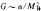The Weinberg-Salam model makes use of three intermediate bosons—two charged bosons, W+ and W-, and one neutral boson, Z0. According to calculations, these particles should be tens of times heavier than the proton: Mw ≳ 40Mp, and Mz≳ 80Mp. The characteristic value of the mass of these bosons is obtained from the value of the weak interaction constant G and the fine-structure constant α = 1/137, which characterizes the electromagnetic interaction of particles (M2w ≳ α/G). The important role of α in the theory reflects the circumstance that the theory is a unified theory of the weak and electromagnetic interactions. The intermediate bosons and neutral currents are needed in order that the theory be consistent.

As W. Heisenberg noted in 1936, the contact interaction of Fermi currents leads to a very strong increase in the weak interaction at short distances. The most recent theoretical investigations have shown that the theory of such an interaction is unrenormalizable: it contains an infinite number of infinite quantities. In contrast to Fermi’s contact theory and a theory with only charged intermediate bosons, a theory making use of symmetrically charged and neutral bosons and currents is renormalizable. It contains only a few infinitely large quantities, which can be eliminated through the operation called renormalization (seeQUANTUM FIELD THEORY).

The intermediate bosons W+, W-, and Z0 are unstable particles. Since their mass is very great, very high energies beyond the capability of present-day accelerators are needed to produce them.

A very important question in the Weinberg-Salam model is that of neutral currents that change strangeness. Such currents have been observed to be suppressed by many orders of magnitude by comparison with charged currents and neutral currents that conserve strangeness. For example, the decay of the long-lived neutral K-meson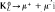is suppressed by comparison with the decay K+vμ by a factor of 108, and the upper limit for the decay K+ → π+ + vμ + ṽμis approximately 10-7 of the total probability of the decay of a K-meson. The experimentally observed small value of the mass difference between the long-lived and short-lived neutral K-mesons attests to an even stronger suppression of neutral currents that change strangeness. This mass difference arises through the transition K0 ⇄ K̃0 and would be very great if there existed a direct interaction of the neutral currents (n̄λ)(nλ̄). In order to explain, within the framework of the theory, the absence of neutral currents that change strangeness, the existence of a fourth quark c, in addition to the three quarks p, n, and λ, has been postulated. This quark is known as the charmed quark. The charged hadronic current interacting with W-bosons in this case has the form

P̄ncos θ + p̄λsin θ + c̄nsin θ + c̄λcos θ

The neutral hadronic current interacting with the Z°-boson transforms quarks_into themselves. This current contains the four terms P̄p, n̄n, λ̄λ̄, and cc and does not contain terms of the type nλ̄. Consequently, it conserves strangeness.

If c-quarks exist, then charmed hadrons, or hadrons containing these quarks, must also exist. In the fall of 1974, American physicists—S. Ting and co-workers and B. Richter and co-workers—detected mesons of masses 3.1 GeV and 3.7 GeV. It is possible that these mesons are states of the type cc. If such an interpretation is correct, then this discovery supports the validity of the strategy underlying the Weinberg-Salam model and the work now being carried out toward a unified theory of the weak, electromagnetic, and strong interactions.

### REFERENCES

Pauli, W. “Narushenie zerkal’noi simmetrii v zakonakh atomnoi fiziki: K staroi i novoi teorii neitrino.” In the collection Teoreticheskaia fizika 20 veka. Moscow, 1962. Pages 376–418.
Wu, C. S., and S. A. Moszkowski. Beta-raspad. Moscow, 1970. (Translated from English.)
Okun’, L. B. Slaboe vzaimodeistvie elementarnykh chastits. Moscow, 1963.
Marshak, R. E., Riazuddin, and C. P. Ryan. Theory of Weak Interactions in Particle Physics. New York, 1969.

L. B. OKUN